Courses

# Classical and Quantum Statistics (Part - 2) - CSIR-NET Physical Sciences Physics Notes | EduRev

## Physics for IIT JAM, UGC - NET, CSIR NET

Created by: Akhilesh Thakur

## Physics : Classical and Quantum Statistics (Part - 2) - CSIR-NET Physical Sciences Physics Notes | EduRev

The document Classical and Quantum Statistics (Part - 2) - CSIR-NET Physical Sciences Physics Notes | EduRev is a part of the Physics Course Physics for IIT JAM, UGC - NET, CSIR NET.
All you need of Physics at this link: Physics

Maxwell velocity distribution

The Maxwell velocity distribution describes the distribution of velocities of the particles of an ideal gas. It will be shown that the Maxwell velocity distribution is of the form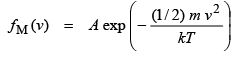(13.30)

where (1/2) m v2 is the kinetic energy of the particles. If the energy of the particles is purely kinetic, the Maxwell distribution can be written as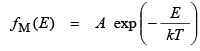(13.31)  The proof of the Maxwell distribution of Eq. (13.30) is conveniently done in two steps. In the first step, the exponential factor is demonstrated, i. e. fM(E) = A exp (– α E). In the second step it is shown that α = 1 / (kT). .

In the theory of ideal gases it is assumed that collisions between particles are elastic. The total energy of two electrons before and after a collision remains the same, that is

E1 + E2 = E1+ E2    (13.32)
where E1 and E2 are the electron energies before the collision and E1 and E2 are the energies after the collision. The probability of a collision of an electron with energy Eand of an electron with energy E2 is proportional to the probability that there is an electron of energy E1 and a second electron with energy E2. If the probability of such a collision is p, then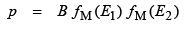(13.33)

where B is a constant. The same consideration is valid for particles with energies E1 and E2. Thus, the probability that two electrons with energies E1 and E2 collide is given by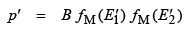(13.34)  If the change in energy before and after the collision is ∆E, then ∆E = E1′ – E1 and ∆E = E2 – E2. Furthermore, if the electron gas is in equilibrium, then p = p′ and one obtains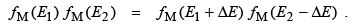(13.35)

Only the exponential function satisfies this condition, that is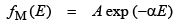(13.36)

where α is a positive yet undetermined constant. The exponent is chosen negative to assure that the occupation probability decreases with higher energies. It will become obvious that α is a universal constant and applies to all carrier systems such as electron-, heavy- or light-hole systems.

Next, the constant α will be determined. It will be shown that α = 1 / kT using the results of the ideal gas theory. The energy of an electron in an ideal gas is given by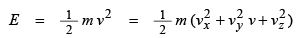(13.37)

The exponential energy distribution of Eq. ( 13.36) and the normalization condition of Eq. (13.15) yield the normalized velocity distribution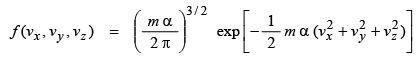(13.38)

The average energy of an electron is obtained by (first) calculating the mean square velocities,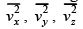from the distribution and (second) using Eq. (13.37) to calculate E from the mean square velocities. One obtains

E = (3 / 2) α −1 . (13.39)

We now use the result from classic gas theory which states according to Eq. (13.28) that the kinetic energy equals E = (3/2) kT. Comparison with Eq. (13.39) yields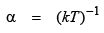(13.40)

which concludes the proof of the Maxwell distribution of Eqs. (13.30) and (13.31).

Having determined the value of α, the explicit form of the normalized maxwellian velocity distribution in cartesian coordinates is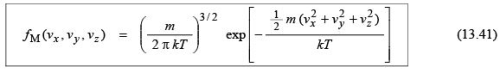Due to the spherical symmetry of the maxwellian velocity distribution, it is useful to express the distribution in spherical coordinates. For the coordinate transformation we note that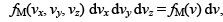, and that a volume element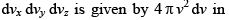in spherical coordinates. The maxwellian velocity distribution in spherical coordinates is then given by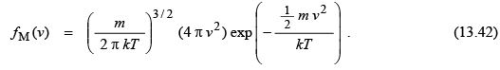The maxwellian velocity distribution is shown in Fig. 13.2. The peak of the distribution, that is the most likely velocity, is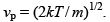. The mean velocity is given by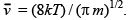. The root-mean-square velocity can only be obtained by numerical integration.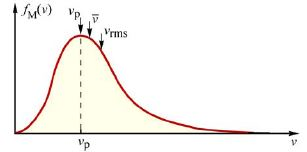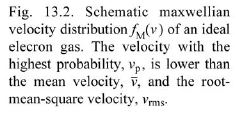The Boltzmann factor

The maxwellian velocity distribution can be changed to an energy distribution by using the substitution E = (1/2) m v2. Noting that the energy interval and the velocity interval are related by dE = m v dv and that the number of electrons in the velocity interval, fM(v) dv, is the same as the number of electrons in the energy interval, fMB(E) dE, then the energy distribution is given by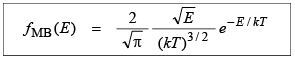(13.43)
which is the Maxwell–Boltzmann distribution.

For large energies, the exponential term in the Maxwell–Boltzmann distribution essentially determines the energy dependence. Therefore, the high-energy approximation of the Maxwell– Boltzmann distribution is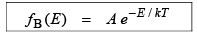(13.44)

which is the Boltzmann distribution. The exponential factor of the distribution, exp (– E / kT), is called the Boltzmann factor or Boltzmann tail. The Boltzmann distribution does not take into account the quantum mechanical properties of an electron gas. The applicability of the distribution is therefore limited to the classical regime, i. e. for E >> kT.

The Fermi–Dirac distribution

In contrast to classical Boltzmann statistics, the quantum mechanical characteristics of an electron gas are taken into account in Fermi–Dirac statistics. The quantum properties which are explicitly taken into account are

• The wave character of electrons. Due to the wave character of electrons the Schrödinger equation has only a finite number of solutions in the energy interval E and E + dE.

• The Pauli principle which states that an eigenstate can be occupied by only two electrons of opposite spin.

Since the Pauli principle strongly restricts the number of carriers per energy level, higher states are populated even at zero temperature. This situation is illustrated in Fig. 13.3, where two electron distributions are illustrated at zero temperature. The distribution in Fig. 13.3(a) does not take into account the Pauli principle while that in Fig. 13.3(b) does.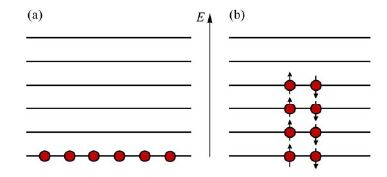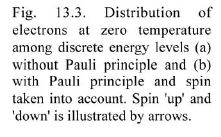The first restriction imposed by quantum mechanics is the finiteness of states within an energy interval E and E + dE. Recall that the finiteness of states played a role in the derivation of the density of states. The density of states in an isotropic semiconductor was shown to be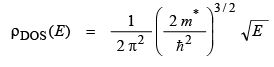(13.45)

where E is the kinetic energy. Note that for the derivation of the density of states the Pauli principle has been taken into account. Therefore, the states given by Eq. (13.45) can be occupied only by one electron. Since the number of states per velocity-interval will be of interest, Eq. (13.45) is modified using E = (1/2) mv2 and dE = mv dv. Note that the number of states per energy interval dE is the same as the number of states per velocity interval dv, i. e. ρDOS(E) dE = ρDOS(v) dv. The number of states per velocity interval (and per unit volume) is then given by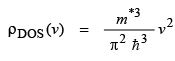(13.46)
for an isotropic semiconductor. The Fermi–Dirac distribution, also called the Fermi distribution, gives the probability that a state of energy E is occupied. Since the Pauli principle has been taken into account in the density of states given by Eq. (13.45), each state can be occupied by at most one electron. The Fermi distribution is given by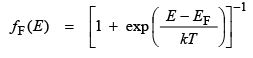(13.47)

where EF is called the Fermi energy. At E = EF the Fermi distribution has a value of 1/2. For small energies the Fermi distribution approaches 1; thus low-energy states are very likely to be populated by electrons. For high energies the Fermi distribution decreases exponentially; states of high energy are less likely to the populated. Particles which follow a Fermi distribution are called fermions. Electrons and holes in semiconductors are such fermions. A system of particles which obey Fermi statistics are called a Fermi gas. Electrons and holes constitute such Fermi gases.

An approximate formula for the Fermi distribution can be obtained for high energies. One obtains for E >> EF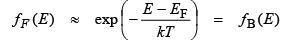(13.48)

This distribution coincides with the Boltzmann distribution. Thus the (quantum-mechanical) Fermi distribution and the (classical) Boltzmann distribution coincide for high energies, i. e. in the classical regime.

Next we prove the Fermi distribution of Eq. (13.47) by considering a collision between two electrons. For simplification we assume that one of the electrons has such a high energy that it belongs to the classical regime of semiconductor statistics. Quantum statistics applies to the other low-energy electron. During the collision of the two electrons, the energy is conserved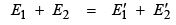(13.49)
where, as before (Eq. 13.32), E1 and E2 are electron energies before the collision and E1 and E2 are the energies after the collision. The probability for the transition (E1, E2) → (E1, E2) is given by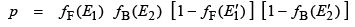(13.50)

where it is assumed that E2 and E2 are relatively large energies and the corresponding electron can be properly described by the Boltzmann distribution. The terms [ 1 – fF(E1) ] and [ 1 – fB(E2) ] describe the probability that the states of energies E1′ and E2 are empty, and are available for the electron after the collision. Further simplification is obtained by considering that E2′ is large and therefore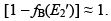. Equation (13.50) then simplifies to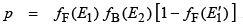(13.51)

The same considerations are valid for the transition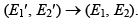. The probability of this transition is given by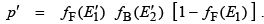(13.52)

Under equilibrium conditions both transition probabilities are the same, i. e. p = p′ . Equating Eqs. (13.51) and (13.52), inserting the Boltzmann distribution for fB(E), and dividing by  fF(E1)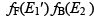yields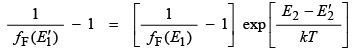(13.53)
which must hold for all E1 and E1. This condition requires that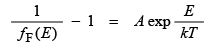(13.54)

where A is a constant. If the value of the constant is taken to be A = exp (– EF / kT) one obtains the Fermi–Dirac distribution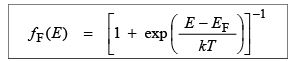(13.54)
which proves Eq. (13.47).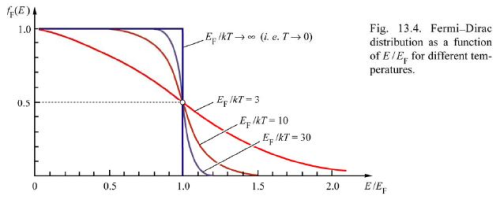The Fermi–Dirac distribution is shown for different temperatures in Fig. 13.4. At the energy E = Ethe probability of a state being populated has always a value of ½ independent of temperature. At higher temperatures, states of higher energies become populated. Note that the Fermi–Dirac distribution is symmetric with respect to EF, that is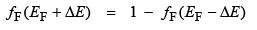(13.55)

where ∆E is any energy measured with respect to the Fermi energy.

The Fermi–Dirac velocity distribution of the particles in a Fermi gas is obtained by multiplication of Eq. (13.46) with Eq. (13.47)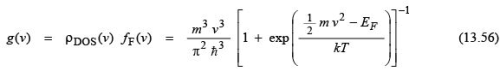where we have used the fact that the energy of the Fermi gas is purely kinetic, i. e. E = (1/2) mv2. Note that g(v) is the number of carriers per velocity interval v and v + dv and per unit volume. If the velocity v is expressed in terms of its components, then the spherical volume element, 4π v2 dv, is modified to a volume element in rectangular coordinates, dvx dvy dvz. Thus, using g(v) dv = g(vx, vy, vz) dvx dvy dvz, one obtains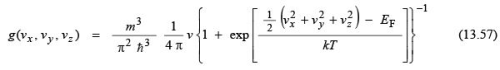which is the Fermi velocity distribution (per unit volume) in cartesian coordinates. The Fermi distribution of energies of an ideal gas is obtained by multiplication of Eq. (13.45) with Eq. (13.47) and is given by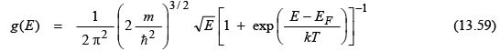when g(E) is the number of particles in the energy interval E and E + dE and per unit volume.

159 docs

,

,

,

,

,

,

,

,

,

,

,

,

,

,

,

,

,

,

,

,

,

;